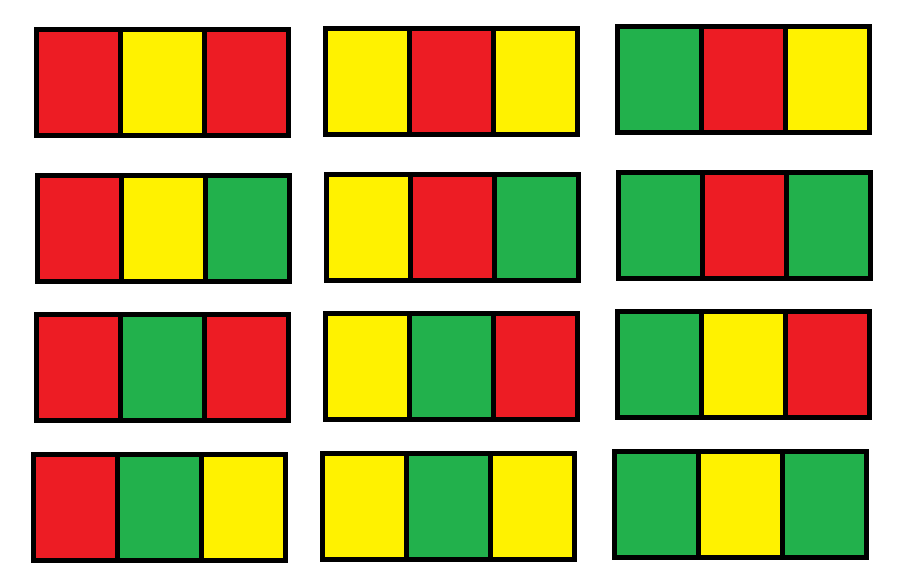##### Welcome to Subscribe On Youtube

Formatted question description: https://leetcode.ca/all/1411.html

# 1411. Number of Ways to Paint N × 3 Grid (Hard)

You have a grid of size n x 3 and you want to paint each cell of the grid with exactly one of the three colours: Red, Yellow or Green while making sure that no two adjacent cells have the same colour (i.e no two cells that share vertical or horizontal sides have the same colour).

You are given n the number of rows of the grid.

Return the number of ways you can paint this grid. As the answer may grow large, the answer must be computed modulo 10^9 + 7.

Example 1:

Input: n = 1
Output: 12
Explanation: There are 12 possible way to paint the grid as shown:Example 2:

Input: n = 2
Output: 54


Example 3:

Input: n = 3
Output: 246


Example 4:

Input: n = 7
Output: 106494


Example 5:

Input: n = 5000
Output: 30228214


Constraints:

• n == grid.length
• grid[i].length == 3
• 1 <= n <= 5000

Related Topics: Dynamic Programming

## Solution 1. DP with State Compression

Once we know the state of the previous row and the row number, the number of the ways for the rest of the rows are fixed.

So we just need to store the mapping from the state to the number of ways.

// OJ: https://leetcode.com/problems/number-of-ways-to-paint-n-3-grid/
// Time: O(N)
// Space: O(N)
// https://leetcode.com/problems/number-of-ways-to-paint-n-3-grid/discuss/574912/JavaC%2B%2B-DFS-Memoization-with-Picture-Clean-code
class Solution {
int memo = {0}, mod = 1e9+7;
int dp(int n, int a, int b, int c) {
if (n == 0) return 1;
if (memo[n][a][b][c]) return memo[n][a][b][c];
int ans = 0, colors = {1, 2, 3};
for (int aa : colors) {
if (aa == a) continue;
for (int bb : colors) {
if (bb == b || bb == aa) continue;
for (int cc : colors) {
if (cc == c || cc == bb) continue;
ans = (ans + dp(n - 1, aa, bb, cc)) % mod;
}
}
}
return memo[n][a][b][c] = ans;
}
public:
int numOfWays(int n) {
return dp(n, 0, 0, 0);
}
};


## Solution 2. DP

// OJ: https://leetcode.com/problems/number-of-ways-to-paint-n-3-grid/
// Time: O(N)
// Space: O(1)
// Ref: https://leetcode.com/problems/number-of-ways-to-paint-n-3-grid/discuss/574943/Java-Detailed-Explanation-with-Graph-Demo-DP-Easy-Understand
class Solution {
typedef long long LL;
public:
int numOfWays(int n) {
LL mod = 1e9+7, color2 = 6, color3 = 6;
for (int i = 2; i <= n; ++i) {
LL c3 = (2 * color3 + 2 * color2) % mod;
LL c2 = (2 * color3 + 3 * color2) % mod;
color2 = c2, color3 = c3;
}
return (color2 + color3) % mod;
}
};


## Solution 3. DP with Matrix Multiplication

// OJ: https://leetcode.com/problems/number-of-ways-to-paint-n-3-grid/
// Time: O(logN)
// Space: O(1)
// Ref: https://leetcode.com/problems/number-of-ways-to-paint-n-3-grid/discuss/575485/C%2B%2BPython-O(logN)-Time
class Solution {
typedef long long LL;
int mod = 1e9+7;
vector<vector<LL>> mul(vector<vector<LL>> &A, vector<vector<LL>> &B) {
int N = A.size(), L = B.size(), M = B.size();
vector<vector<LL>> C(N, vector<LL>(M));
for (int i = 0; i < N; ++i) {
for (int j = 0; j < M; ++j) {
for (int k = 0; k < L; ++k) {
C[i][j] = (C[i][j] + A[i][k] * B[k][j]) % mod;
}
}
}
return C;
}
public:
int numOfWays(int n) {
vector<vector<LL>> ans = { {6, 6} }, M = { {3, 2}, {2, 2} };
for (--n; n; n >>= 1, M = mul(M, M)) {
if (n % 2) ans = mul(ans, M);
}
return (ans + ans) % mod;
}
};

• class Solution {
public int numOfWays(int n) {
final int MODULO = 1000000007;
long prev2 = 6, prev3 = 6;
for (int i = 2; i <= n; i++) {
long curr2 = (prev2 * 3 + prev3 * 2) % MODULO;
long curr3 = (prev2 * 2 + prev3 * 2) % MODULO;
prev2 = curr2;
prev3 = curr3;
}
int ways = (int) ((prev2 + prev3) % MODULO);
return ways;
}
}

############

class Solution {
public int numOfWays(int n) {
int mod = (int) 1e9 + 7;
long f0 = 6, f1 = 6;
for (int i = 0; i < n - 1; ++i) {
long g0 = (3 * f0 + 2 * f1) % mod;
long g1 = (2 * f0 + 2 * f1) % mod;
f0 = g0;
f1 = g1;
}
return (int) (f0 + f1) % mod;
}
}

• // OJ: https://leetcode.com/problems/number-of-ways-to-paint-n-3-grid/
// Time: O(N)
// Space: O(N)
// https://leetcode.com/problems/number-of-ways-to-paint-n-3-grid/discuss/574912/JavaC%2B%2B-DFS-Memoization-with-Picture-Clean-code
class Solution {
int memo = {0}, mod = 1e9+7;
int dp(int n, int a, int b, int c) {
if (n == 0) return 1;
if (memo[n][a][b][c]) return memo[n][a][b][c];
int ans = 0, colors = {1, 2, 3};
for (int aa : colors) {
if (aa == a) continue;
for (int bb : colors) {
if (bb == b || bb == aa) continue;
for (int cc : colors) {
if (cc == c || cc == bb) continue;
ans = (ans + dp(n - 1, aa, bb, cc)) % mod;
}
}
}
return memo[n][a][b][c] = ans;
}
public:
int numOfWays(int n) {
return dp(n, 0, 0, 0);
}
};

• class Solution:
def numOfWays(self, n: int) -> int:
mod = 10**9 + 7
f0 = f1 = 6
for _ in range(n - 1):
g0 = (3 * f0 + 2 * f1) % mod
g1 = (2 * f0 + 2 * f1) % mod
f0, f1 = g0, g1
return (f0 + f1) % mod


• func numOfWays(n int) int {
mod := int(1e9) + 7
f0, f1 := 6, 6
for n > 1 {
n--
g0 := (f0*3 + f1*2) % mod
g1 := (f0*2 + f1*2) % mod
f0, f1 = g0, g1
}
return (f0 + f1) % mod
}